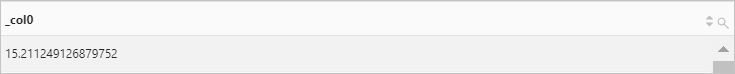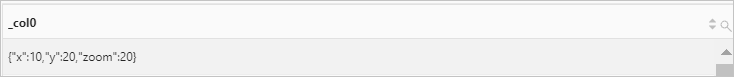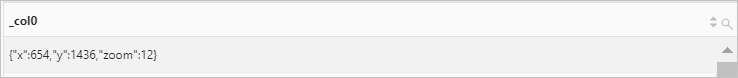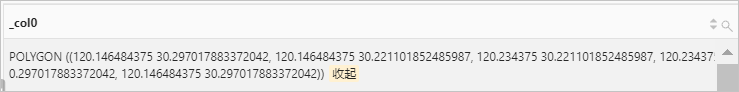POINT (0 0)

## 函数列表

ST_GeometryFromText函数 ST_GeometryFromText(x) 根据输入的WKT文本构造一个空间几何体。
ST_LineFromText函数 ST_LineFromText(x) 根据输入的WKT文本构造一条线段。
ST_Polygon函数 ST_Polygon(x) 根据输入的WKT文本构造一个多边形。
ST_Point函数 ST_Point(x, y) 根据输入的WKT文本构造一个点。

ST_Buffer函数 ST_Buffer(x, distance) 返回距离指定空间几何体一定距离的空间几何体。
ST_Difference函数 ST_Difference(x, y) 返回两个空间几何体不同点的集合。
ST_Envelope函数 ST_Envelope(x) 返回空间几何体的最小边界框。
ST_ExteriorRing函数 ST_ExteriorRing(x) 返回空间几何体的外环（线段形式）。
ST_Intersection函数 ST_Intersection(x, y) 返回两个空间几何体的交集点。
ST_SymDifference函数 ST_SymDifference(x, y) 返回两个空间几何体的不同点，然后组成一个新的空间几何体。

ST_Crosses函数 ST_Crosses(x, y) 判断两个空间几何体是否存在相同的内部点。如果存在，则返回true。
ST_Disjoint函数 ST_Disjoint(x, y) 判断两个空间几何体是否没有任何交集。 如果没有，则返回true。
ST_Equals函数 ST_Equals(x, y) 判断两个空间几何体是否完全相同。如果是，则返回true。
ST_Intersects函数 ST_Intersects(x, y) 判断两个空间几何体的平面投影是否存在共同点。如果是，则返回true。
ST_Overlaps函数 ST_Overlaps(x, y) 判断两个空间几何体的维度是否相同。如果两个空间几何体的维度相同且不是包含关系，则返回true。
ST_Relate函数 ST_Relate(x, y, patternMatrix string) 判断两个空间几何体是否相关。如果是，则返回true。
ST_Touches函数 ST_Touches(x, y) 判断两个空间几何体是否只有边界存在关联，没有共同的内部点。如果是，则返回true。
ST_Within函数 ST_Within(x, y) 判断第一个空间几何体是否完全在第二个空间几何体内部（边界无交集）。如果是，则返回true。
Accessors ST_Area函数 ST_Area(x) 使用欧几里得测量法计算空间几何体在二维平面上的投影面积。
ST_Centroid函数 ST_Centroid(x) 返回空间几何实体的中心点。
ST_CoordDim函数 ST_CoordDim(x) 返回空间几何体的坐标维度。
ST_Dimension函数 ST_Dimension(x) 返回空间几何实体的固有维度，必须小于或等于坐标维度。
ST_Distance函数 ST_Distance(x, y) 计算两个空间几何体之间的最小距离。
ST_EndPoint函数 ST_EndPoint(x) 返回线段中的最后一个点。
ST_IsClosed函数 ST_IsClosed(x) 判断输入的空间几何体是否封闭。如果是，则返回true。
ST_IsEmpty函数 ST_IsEmpty(x) 判断输入的空间几何体是否为空。如果是，则返回true。
ST_IsRing函数 ST_IsRing(x) 判断输入的空间几何体是否为闭合的简单线段（环）。如果是，则返回true。
ST_Length函数 ST_Length(x) 使用欧几里得测量法计算线段的二维投影长度。如果存在多条线段，则返回所有线段的长度之和。
ST_NumPoints函数 ST_NumPoints(x) 返回空间几何体中点的个数。
ST_NumInteriorRing函数 ST_NumInteriorRing(x) 计算空间几何体中内部环的数量。
ST_StartPoint函数 ST_StartPoint(x) 返回线段中的第一个点。
ST_X函数 ST_X(x) 返回输入点的第一个X轴坐标。
ST_XMax函数 ST_XMax(x) 返回空间几何体的第一个最大的X轴坐标。
ST_XMin函数 ST_XMin(x) 返回空间几何体的第一个最小的X轴坐标。
ST_Y函数 ST_Y(x) 返回输入点的第一个Y轴坐标。
ST_YMax函数 ST_YMax(x) 返回空间几何体的第一个最大的Y轴坐标。
ST_YMin函数 ST_YMin(x) 返回几何体的第一个最小的Y轴坐标。
Bing图块 bing_tile函数 bing_tile(x, y, zoom_level) 通过X坐标、Y坐标和缩放级别构造一个Bing图块。
bing_tile_at函数 bing_tile_at(x, y, zoom_level) 通过经纬度和缩放级别构造一个Bing图块。
bing_tile_coordinates函数 bing_tile_coordinates(x) 返回目标Bing图块对应的X坐标和Y坐标。
bing_tile_polygon函数 bing_tile_polygon(x) 返回目标Bing图块的多边形格式。
bing_tile_zoom_level函数 bing_tile_zoom_level(x) 返回目标Bing图块的缩放级别。

## ST_AsText函数

ST_AsText函数用于将一个空间几何体转变成WKT文本。

### 语法

``ST_AsText(x)``

### 参数说明

x 参数值为geometry类型。

varchar类型。

### 示例

• 查询和分析语句
``* | SELECT ST_AsText(ST_Point(1,1))``
• 查询和分析结果## ST_GeometryFromText函数

ST_GeometryFromText函数会根据您输入的WKT文本构造一个空间几何体。

### 语法

``ST_GeometryFromText(x)``

x 参数值为varchar类型。

geometry类型。

### 示例

• 查询和分析语句
``* | SELECT ST_GeometryFromText('multipolygon(((10 10,10 20,20 20,20 15,10 10), (50 40,50 50,60 50,60 40,50 40)))')``
• 查询和分析结果## ST_LineFromText函数

ST_LineFromText函数会根据您输入的WKT文本构造一条线段。

### 语法

``ST_LineFromText(x)``

x 参数值为varchar类型。

linestring类型。

### 示例

• 查询和分析语句
``* | SELECT ST_LineFromText('linestring(10 10,20 20)')``
• 查询和分析结果## ST_Polygon函数

ST_Polygon函数会根据您输入的WKT文本构造一个多边形。

### 语法

``ST_Polygon(x)``

x 参数值为varchar类型。

polygon类型。

### 示例

• 查询和分析语句
``* | SELECT ST_Polygon('polygon((10 10,10 20,20 20,20 15,10 10))')``
• 查询和分析结果## ST_Point函数

ST_Point函数会根据您输入的WKT文本构造一个点。

### 语法

``ST_Point(x, y)``

### 参数说明

x 参数值为geometry类型。
y 参数值为geometry类型。

point类型。

### 示例

• 查询和分析语句
``* | SELECT ST_Point(0,0)``
• 查询和分析结果## ST_Boundary函数

ST_Boundary函数用于返回空间几何体的边界。
• 点的边界为空，即返回POINT EMPTY。
• 线段的边界由线段的端点组成。
• 多边形的边界由构成多边形的外环及其所有内环的线段组成。

### 语法

``ST_Boundary(x)``

### 参数说明

x 参数值为geography类型。

geography类型。

### 示例

• 查询和分析语句
``* | SELECT  ST_Boundary(ST_Polygon('polygon((10 10,10 20,20 20,20 15,10 10))'))``
• 查询和分析结果## ST_Buffer函数

ST_Buffer函数用于返回距离指定空间几何体一定距离的空间几何体。

### 语法

``ST_Buffer(x, distance)``

### 参数说明

x 参数值为geometry类型。
distance 距离。

geometry类型。

### 示例

• 查询和分析语句
``* | SELECT ST_Buffer(ST_Point(1,1),1)``
• 查询和分析结果## ST_Difference函数

ST_Difference函数用于返回两个空间几何体不同点的集合。

### 语法

``ST_Difference(x, y)``

### 参数说明

x 参数值为geometry类型。
y 参数值为geometry类型。

geometry类型。

### 示例

• 查询和分析语句
``````* |
SELECT
ST_Difference(
ST_GeometryFromText(
'multipolygon (((10 10,10 20,20 20,0 15,0 10), (50 40,50 50,60 50,60 40,50 40)))'
),
ST_GeometryFromText(
'multipolygon (((10 10,10 20,20 20,20 15,10 10), (50 40,50 50,60 50,60 40,50 50)))'
)
) AS "Difference"``````
• 查询和分析结果## ST_Envelope函数

ST_Envelope函数用于返回空间几何体的最小边界框。

### 语法

``ST_Envelope(x)``

### 参数说明

x 参数值为geometry类型。

geometry类型。

### 示例

• 查询和分析语句
``````* |
SELECT
ST_Envelope(
ST_GeometryFromText(
'multipolygon (((10 10,10 20,20 20,20 15,10 10), (50 40,50 50,60 50,60 40,50 40)))'
)
) ``````
• 查询和分析结果## ST_ExteriorRing函数

ST_ExteriorRing函数用于返回空间几何体的外环（线段形式）。

### 语法

``ST_ExteriorRing(x)``

### 参数说明

x 参数值为geometry类型。

geometry类型。

### 示例

• 查询和分析语句
``````* |
SELECT
ST_ExteriorRing(
ST_GeometryFromText(
'multipolygon (((10 10,10 20,20 20,20 15,10 10), (50 40,50 50,60 50,60 40,50 40)))'
)
)``````
• 查询和分析结果## ST_Intersection函数

ST_Intersection函数用于返回两个空间几何体的交集点。

### 语法

``ST_Intersection(x, y)``

### 参数说明

x 参数值为geometry类型。
y 参数值为geometry类型。

geometry类型。

### 示例

• 查询和分析语句
``````* |
SELECT
ST_Intersection(
ST_GeometryFromText(
'multipolygon (((10 10,10 20,20 20,20 15,10 10), (50 40,50 50,60 50,60 40,50 40)))'
),
ST_GeometryFromText(
'multipolygon (((10 10,10 20,20 20,20 15,10 10), (50 40,50 50,60 50,60 40,50 50)))'
)
) ``````
• 查询和分析结果## ST_SymDifference函数

ST_SymDifference函数用于返回两个空间几何体的不同点，然后组成一个新的空间几何体。

### 语法

``ST_SymDifference(x, y)``

### 参数说明

x 参数值为geometry类型。
y 参数值为geometry类型。

geometry类型。

### 示例

• 查询和分析语句
``````* |
SELECT
ST_SymDifference(
ST_GeometryFromText(
'multipolygon (((10 10,10 20,20 20,20 15,10 10), (50 40,50 50,60 50,60 40,50 40)))'
),
ST_GeometryFromText(
'multipolygon (((10 10,10 20,20 20,20 15,10 10), (50 40,50 50,60 50,60 40,50 50)))'
)
)``````
• 查询和分析结果## ST_Contains函数

ST_Contains函数用于判断第一个空间几何体是否包含第二个空间几何体（边界可存在交集）。如果包含，则返回true。

### 语法

``ST_Contains(x, y)``

### 参数说明

x 参数值为geometry类型。
y 参数值为geometry类型。

boolean类型。

### 示例

• 查询和分析语句
``````* |
SELECT
ST_Contains(
ST_GeometryFromText(
'polygon((10 10,10 20,20 20,20 15,10 10))'
),
ST_GeometryFromText(
'point(11 11)'
)
)``````
• 查询和分析结果## ST_Crosses函数

ST_Crosses函数用于判断两个空间几何体是否存在相同的内部点。如果存在，则返回true。

### 语法

``ST_Crosses(x, y)``

### 参数说明

x 参数值为geometry类型。
y 参数值为geometry类型。

boolean类型。

### 示例

• 查询和分析语句
``````* |
SELECT
ST_Crosses(
ST_GeometryFromText(
'multipolygon (((10 10, 10 20, 20 20, 20 15 , 10 10), (50 40, 50 50, 60 50, 60 40, 50 40)))'
),
ST_GeometryFromText(
'multipolygon (((10 10, 10 20, 20 20, 20 15 , 10 10), (50 40, 50 50, 60 50, 60 40, 50 50)))'
)
)``````
• 查询和分析结果## ST_Disjoint函数

ST_Disjoint函数用于判断两个空间几何体是否没有任何交集。 如果没有，则返回true。

### 语法

``ST_Disjoint(x, y)``

### 参数说明

x 参数值为geometry类型。
y 参数值为geometry类型。

boolean类型。

### 示例

• 查询和分析语句
``````* |
SELECT
ST_Disjoint(
ST_GeometryFromText(
'multipolygon (((10 10,10 20,20 20,20 15,10 10), (50 40,50 50,60 50,60 40,50 40)))'
),
ST_GeometryFromText(
'multipolygon (((10 10,10 20,20 20,20 15,10 10), (50 40,50 50,60 50,60 40,50 50)))'
)
)``````
• 查询和分析结果## ST_Equals函数

ST_Equals函数用于判断两个空间几何体是否完全相同。如果是，则返回true。

### 语法

``ST_Equals(x, y)``

### 参数说明

x 参数值为geometry类型。
y 参数值为geometry类型。

boolean类型。

### 示例

• 查询和分析语句
``````* |
SELECT
ST_Equals(
ST_GeometryFromText(
'multipolygon(((10 10,10 20,20 20,20 15,10 10),(50 40,50 50,60 50,60 40,50 40)))'
),
ST_GeometryFromText(
'multipolygon(((10 10,10 20,20 20,20 15,10 10),(50 40,50 50,60 50,60 40,50 50)))'
)
)``````
• 查询和分析结果## ST_Intersects函数

ST_Intersects函数用于判断两个空间几何体的平面投影是否存在共同点。如果是，则返回true。

### 语法

``ST_Intersects(x, y)``

### 参数说明

x 参数值为geometry类型。
y 参数值为geometry类型。

boolean类型。

### 示例

• 查询和分析语句
``````* |
SELECT
ST_Intersects(
ST_GeometryFromText(
'multipolygon (((10 10,10 20,20 20,20 15,10 10), (50 40,50 50,60 50,60 40,50 40)))'
),
ST_GeometryFromText(
'multipolygon (((10 10,10 20,20 20,20 15,10 10), (50 40,50 50,60 50,60 40,50 50)))'
)
)``````
• 查询和分析结果## ST_Overlaps函数

ST_Overlaps函数用于判断两个空间几何体的维度是否相同。如果两个空间几何体的维度相同且不是包含关系，则返回true。

### 语法

``ST_Overlaps(x, y)``

### 参数说明

x 参数值为geometry类型。
y 参数值为geometry类型。

boolean类型。

### 示例

• 查询和分析语句
``````* |
SELECT
ST_Overlaps(
ST_GeometryFromText(
'multipolygon (((10 10,10 20,20 20,20 15,10 10), (50 40,50 50,60 50,60 40,50 40)))'
),
ST_GeometryFromText(
'multipolygon (((10 10,10 20,20 20,20 15,10 10), (50 40,50 50,60 50,60 40,50 50)))'
)
)``````
• 查询和分析结果## ST_Relate函数

ST_Relate函数用于判断两个空间几何体是否相关（内部或边界以任何方式相关）。如果是，则返回true。

### 语法

``ST_Relate(x, y, patternMatrix string)``

### 参数说明

x 参数值为geometry类型。
y 参数值为geometry类型。
patternMatrix string DE-9IM模式矩阵字符串，参数值为varchar类型。

boolean类型。

### 示例

• 查询和分析语句
``````* |
SELECT
ST_Relate(
ST_GeometryFromText(
'multipolygon (((10 10,10 20,20 20,20 15,10 10), (50 40,50 50,60 50,60 40,50 40)))'
),
ST_GeometryFromText(
'multipolygon (((10 10,10 20,20 20,20 15,10 10), (50 40,50 50,60 50,60 40,50 50)))'
),  '****T****'
)``````
• 查询和分析结果## ST_Touches函数

ST_Touches函数用于判断两个空间几何体是否只有边界存在关联，没有共同的内部点。如果是，则返回true。

### 语法

``ST_Touches(x, y)``

### 参数说明

x 参数值为geometry类型。
y 参数值为geometry类型。

boolean类型。

### 示例

• 查询和分析语句
``````* |
SELECT
ST_Touches(
ST_GeometryFromText(
'multipolygon (((10 10,10 20,20 20,20 15,10 10), (50 40,50 50,60 50,60 40,50 40)))'
),
ST_GeometryFromText(
'multipolygon (((10 10,10 20,20 20,20 15,10 10), (50 40,50 50,60 50,60 40,50 50)))'
)
)``````
• 查询和分析结果## ST_Within函数

ST_Within函数用于判断第一个空间几何体是否完全在第二个空间几何体内部（边界无交集）。如果是，则返回true。

### 语法

``ST_Within(x, y)``

### 参数说明

x 参数值为geometry类型。
y 参数值为geometry类型。

boolean类型。

### 示例

• 查询和分析语句
``````* |
SELECT
ST_Within(
ST_GeometryFromText(
'multipolygon (((10 10,10 20,20 20,20 15,10 10), (50 40,50 50,60 50,60 40,50 40)))'
),
ST_GeometryFromText(
'multipolygon (((10 10,10 20,20 20,20 15,10 10), (50 40,50 50,60 50,60 40,50 50)))'
)
)``````
• 查询和分析结果## ST_Area函数

ST_Area函数使用欧几里得测量法计算空间几何体在二维平面上的投影面积。

### 语法

``ST_Area(x)``

### 参数说明

x 参数值为geometry类型。

double类型。

### 示例

• 查询和分析语句
``````* |
SELECT
ST_Area(
ST_GeometryFromText(
'multipolygon (((10 10,10 20,20 20,20 15,10 10), (50 40,50 50,60 50,60 40,50 40)))'
)
)``````
• 查询和分析结果## ST_Centroid函数

ST_Centroid函数用于返回空间几何体的中心点。

### 语法

``ST_Centroid(x)``

### 参数说明

x 参数值为geometry类型。

geometry类型。

### 示例

• 查询和分析语句
``````* |
SELECT
ST_Centroid(
ST_GeometryFromText(
'multipolygon (((10 10,10 20,20 20,20 15,10 10), (50 40,50 50,60 50,60 40,50 40)))'
)
)``````
• 查询和分析结果## ST_CoordDim函数

ST_CoordDim函数用于返回空间几何体的坐标维度。

### 语法

``ST_CoordDim(x)``

### 参数说明

x 参数值为geometry类型。

bigint类型。

### 示例

• 查询和分析语句
``````* |
SELECT
ST_CoordDim(
ST_GeometryFromText(
'multipolygon (((10 10,10 20,20 20,20 15,10 10), (50 40,50 50,60 50,60 40,50 40)))'
)
)``````
• 查询和分析结果## ST_Dimension函数

ST_Dimension函数用于返回空间几何体的固有维度，必须小于或等于坐标维度。

### 语法

``ST_Dimension(x)``

### 参数说明

x 参数值为geometry类型。
• x为点或空的空间几何体时，返回值为0。
• x为线段时，返回值为1。
• x为多边形时，返回值为2。
• x为空间几何体时，返回值为集合的最大维度。

bigint类型。

### 示例

• 查询和分析语句
``````* |
SELECT
ST_Dimension(
ST_GeometryFromText(
'multipolygon (((10 10,10 20,20 20,20 15,10 10), (50 40,50 50,60 50,60 40,50 40)))'
)
)``````
• 查询和分析结果## ST_Distance函数

ST_Distance函数用于计算两个空间几何体之间的最小距离。

### 语法

``ST_Distance(x, y)``

### 参数说明

x 参数值为geometry类型。
y 参数值为geometry类型。

double类型。

### 示例

• 查询和分析语句
``````* |
SELECT
ST_Distance(
ST_GeometryFromText(
'multipolygon (((10 10,10 20,20 20,20 15,10 10), (50 40,50 50,60 50,60 40,50 50)))'
),
ST_GeometryFromText(
'multipolygon (((10 10,10 20,20 20,20 15,10 10), (50 40,50 50,60 50,60 40,50 40)))'
)
)``````
• 查询和分析结果## ST_EndPoint函数

ST_EndPoint函数用于返回线段中的最后一个点。

### 语法

``ST_EndPoint(x)``

### 参数说明

x 参数值为geometry类型。

point类型。

### 示例

• 查询和分析语句
``````* |
SELECT
ST_EndPoint(
ST_LineFromText(
'linestring (10 10,20 20)'
)
)``````
• 查询和分析结果## ST_IsClosed函数

ST_IsClosed函数用于判断空间几何体是否封闭。如果是，则返回true。

### 语法

``ST_IsClosed(x)``

### 参数说明

x 参数值为geometry类型。

boolean类型。

### 示例

• 查询和分析语句
``````* |
SELECT
ST_IsClosed(
ST_LineFromText(
'linestring (10.05 10.28 , 20.95 20.89 )'
)
)``````
• 查询和分析结果## ST_IsEmpty函数

ST_IsEmpty函数用于判断输入的空间几何体是否为空。如果是，则返回true。

### 语法

``ST_IsEmpty(x)``

### 参数说明

x 参数值为geometry类型。

boolean类型。

### 示例

• 查询和分析语句
``* | SELECT ST_IsEmpty(ST_Point(1,1))``
• 查询和分析结果## ST_IsRing函数

ST_IsRing函数用于判断输入的空间几何体是否为闭合的简单线段（环）。如果是，则返回true。

### 语法

``ST_IsRing(x)``

### 参数说明

x 参数值为geometry类型。

boolean类型。

### 示例

• 查询和分析语句
``````* |
SELECT
ST_IsRing(
ST_LineFromText(
'linestring (10.05 10.28,20.95 20.89 )'
)
)``````
• 查询和分析结果## ST_Length函数

ST_Length函数使用欧几里得测量法计算线段的二维投影长度。如果存在多条线段，则返回所有线段的长度之和。

### 语法

``ST_Length(x)``

### 参数说明

x 参数值为geometry类型。

double类型。

### 示例

• 查询和分析语句
``````* |
SELECT
ST_Length(
ST_LineFromText(
'linestring (10.05 10.28,20.95 20.89)'
)
)``````
• 查询和分析结果## ST_NumPoints函数

ST_NumPoints函数用于返回空间几何体中点的个数。

### 语法

``ST_NumPoints(x)``

### 参数说明

x 参数值为geometry类型。

bigint类型。

### 示例

• 查询和分析语句
``````* |
SELECT
ST_NumPoints(
ST_LineFromText('linestring (10 10,20 20)')
)``````
• 查询和分析结果## ST_NumInteriorRing函数

ST_NumInteriorRing函数用于计算空间几何体中内部环的数量。

### 语法

``ST_NumInteriorRing(x)``

### 参数说明

x 参数值为geometry类型。

bigint类型。

### 示例

• 查询和分析语句
``````* |
SELECT
ST_NumInteriorRing(
ST_GeometryFromText(
'multipolygon (((10 10,10 20,20 20,20 15,10 10), (50 40,50 50,60 50,60 40,50 40)))'
)
)``````
• 查询和分析结果## ST_StartPoint函数

ST_StartPoint函数用于返回线段中的第一个点。

### 语法

``ST_StartPoint(x)``

### 参数说明

x 参数值为geometry类型。

point类型。

### 示例

• 查询和分析语句
``````* |
SELECT
ST_StartPoint(
ST_LineFromText(
'linestring (10 10,20 20 )'
)
)``````
• 查询和分析结果## ST_X函数

ST_X函数用于返回输入点的X轴坐标。

### 语法

``ST_X(x)``

x 参数值为point类型。

double类型。

### 示例

• 查询和分析语句
``* | SELECT ST_X(ST_Point(1,3))``
• 查询和分析结果## ST_XMax函数

ST_XMax函数用于返回空间几何体的第一个最大的X轴坐标。

### 语法

``ST_XMax(x)``

### 参数说明

x 参数值为geometry类型。

double类型。

### 示例

• 查询和分析语句
``````* |
SELECT
ST_XMax(
ST_GeometryFromText(
'multipolygon (((10 10,10 20,20 20,20 15,10 10), (50 40,50 50,60 50,60 40,50 40)))'
)
)``````
• 查询和分析结果## ST_XMin函数

ST_XMin函数用于返回空间几何体的第一个最小的X轴坐标。

### 语法

``ST_XMin(x)``

### 参数说明

x 参数值为geometry类型。

double类型。

### 示例

• 查询和分析语句
``````* |
SELECT
ST_XMin(
ST_GeometryFromText(
'multipolygon (((10 10,10 20,20 20,20 15,10 10), (50 40,50 50,60 50,60 40,50 40)))'
)
)``````
• 查询和分析结果## ST_Y函数

ST_Y函数用于返回输入点的Y轴坐标。

### 语法

``ST_Y(x)``

x 参数值为point类型。

double类型。

### 示例

• 查询和分析语句
``* | SELECT ST_Y(ST_Point(1,3))``
• 查询和分析结果## ST_YMax函数

ST_YMax函数用于返回空间几何体的第一个最大的Y轴坐标。

### 语法

``ST_YMax(x)``

### 参数说明

x 参数值为geometry类型。

double类型。

### 示例

• 查询和分析语句
``````* |
SELECT
ST_YMax(
ST_GeometryFromText(
'multipolygon (((10 10,10 20,20 20,20 15,10 10), (50 40,50 50,60 50,60 40,50 40)))'
)
)``````
• 查询和分析结果## ST_YMin函数

ST_YMin函数用于返回空间几何体的第一个最小的Y轴坐标。

### 语法

``ST_YMin(x)``

### 参数说明

x 参数值为geometry类型。

double类型。

### 示例

• 查询和分析语句
``````* |
SELECT
ST_YMin(
ST_GeometryFromText(
'multipolygon (((10 10,10 20,20 20,20 15,10 10), (50 40,50 50,60 50,60 40,50 40)))'
)
)``````
• 查询和分析结果## bing_tile函数

bing_tile函数用于构造一个Bing图块。

### 语法

• 通过X坐标、Y坐标和缩放级别构造一个Bing图块。
``bing_tile(x, y, zoom_level)``
• 通过四叉树键构造一个Bing图块。
``bing_tile(quadKey)``

### 参数说明

x X坐标，参数值为integer类型。
y Y坐标，参数值为integer类型。
zoom_level 缩放级别，取值范围为[1,23]，参数值为integer类型。

BingTile类型。

### 示例

• 示例1：通过X坐标、Y坐标和缩放级别构造一个Bing图块。
• 查询和分析语句
``* | SELECT bing_tile(10, 20, 20)``
• 查询和分析结果• 示例2：通过四叉树键构造一个Bing图块。
• 查询和分析语句
``* | SELECT bing_tile(bing_tile_quadkey(bing_tile(10, 20, 20)))``
• 查询和分析结果## bing_tile_at函数

bing_tile_at函数通过经纬度和缩放级别构造一个Bing图块。

### 语法

``bing_tile_at(x, y, zoom_level)``

### 参数说明

x 纬度，取值范围为[-85.05112878,85.05112878]，参数值为double类型。
y 经度，取值范围为[-180,180]，参数值为double类型。
zoom_level 缩放级别，取值范围为[1,23]，参数值为integer类型。

BingTile类型。

### 示例

• 查询和分析语句
``* | SELECT bing_tile_at(47.265511, -122.465691, 12)``
• 查询和分析结果## bing_tile_coordinates函数

bing_tile_coordinates函数用于返回目标Bing图块对应的X坐标和Y坐标。

### 语法

``bing_tile_coordinates(x)``

### 参数说明

x 参数值为BingTile类型。

### 返回值类型

array(integer,integer)类型。

### 示例

• 查询和分析语句
``* | SELECT bing_tile_coordinates(bing_tile_at(47.265511, -122.465691, 12))``
• 查询和分析结果## bing_tile_polygon函数

bing_tile_polygon函数用于返回目标Bing图块的多边形格式。

### 语法

``bing_tile_polygon(x)``

### 参数说明

x 参数值为BingTile类型。

polygon类型。

### 示例

• 查询和分析语句
``* | SELECT bing_tile_polygon(bing_tile_at(30.26, 120.19, 12))``
• 查询和分析结果### 语法

``bing_tile_quadkey(x)``

### 参数说明

x 参数值为BingTile类型。

varchar类型。

### 示例

• 查询和分析语句
``* | SELECT bing_tile_quadkey(bing_tile(10, 20, 20))``
• 查询和分析结果## bing_tile_zoom_level函数

bing_tile_zoom_level函数用于返回目标Bing图块的缩放级别。

### 语法

``bing_tile_zoom_level(x)``

### 参数说明

x 参数值为BingTile类型。

double类型。

### 示例

• 查询和分析语句
``* | SELECT bing_tile_zoom_level(bing_tile(10, 20, 20))``
• 查询和分析结果